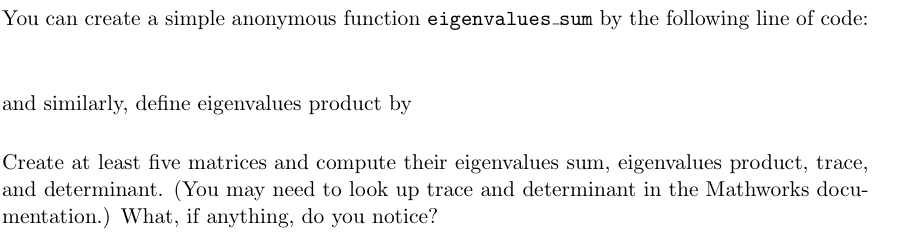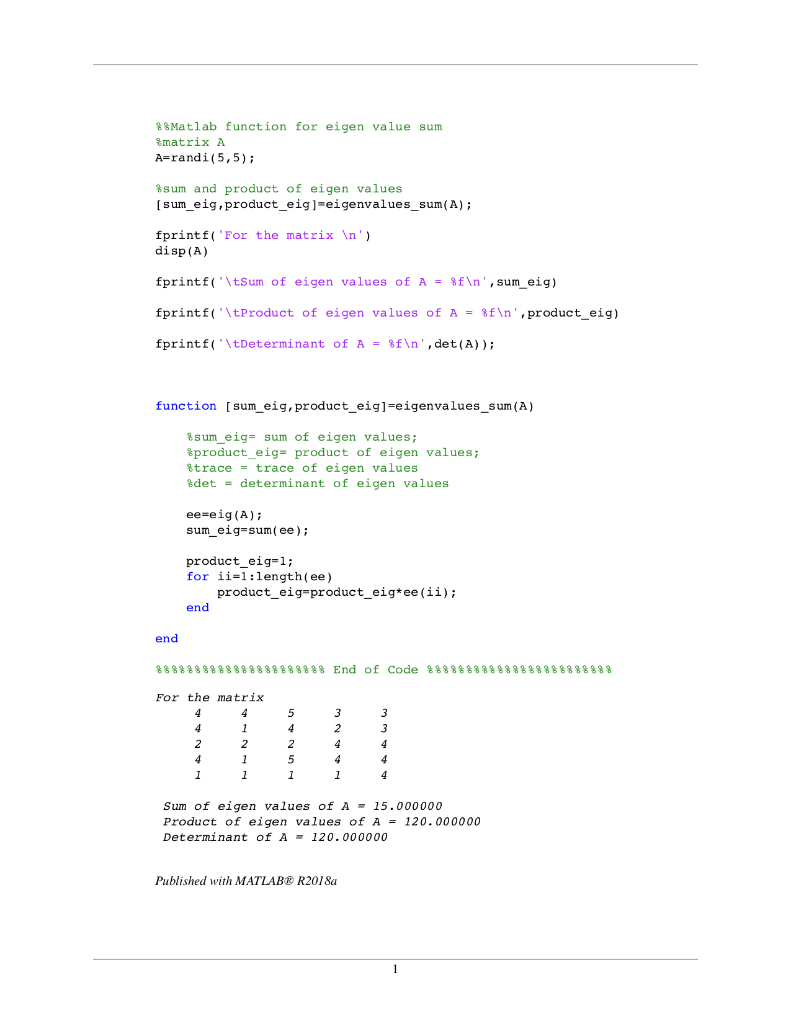Homework Help Question & Answers

# You can create a simple anonymous function eigenvalues sum by the following line of code: and similarly, define eigenvalues product by Create at least five matrices and compute their eigenvalues sum,...You can create a simple anonymous function

eigenvalues

sum

by the following line of code:

and similarly, define eigenvalues product by

Create at least five matrices and compute their eigenvalues sum, eigenvalues product, trace,

and determinant. (You may need to look up trace and determinant in the Mathworks docu-

mentation.) What, if anything, do you notice?

MATLAB: I need the code for everything in the picture, not just the bottom paragraph. Thanks!

You can create a simple anonymous function eigenvalues sum by the following line of code and similarly, define eigenvalues product by Create at least five matrices and compute their eigenvalues sum, eigenvalues product, trace, and determinant. (You may need to look up trace and determinant in the Mathworks docu mentation.) What, if anything, do you notice?%%Matlab function for eigen value sum
%matrix A
A=randi(5,5);

%sum and product of eigen values
[sum_eig,product_eig]=eigenvalues_sum(A);

fprintf('For the matrix \n')
disp(A)

fprintf('\tSum of eigen values of A = %f\n',sum_eig)

fprintf('\tProduct of eigen values of A = %f\n',product_eig)

fprintf('\tDeterminant of A = %f\n',det(A));

function [sum_eig,product_eig]=eigenvalues_sum(A)

%sum_eig= sum of eigen values;
%product_eig= product of eigen values;
%trace = trace of eigen values
%det = determinant of eigen values

ee=eig(A);
sum_eig=sum(ee);

product_eig=1;
for ii=1:length(ee)
product_eig=product_eig*ee(ii);
end

end

%%%%%%%%%%%%%%%%%%%%%% End of Code %%%%%%%%%%%%%%%%%%%%%%%%

##### Add Answer of: You can create a simple anonymous function eigenvalues sum by the following line of code: and similarly, define eigenvalues product by Create at least five matrices and compute their eigenvalues sum,...
More Homework Help Questions Additional questions in this topic.

• #### the alkaline-earth metals react similarly because they all have the same number of electrons in their outer energy level

Need Online Homework Help?

Get FREE EXPERT Answers
WITHIN MINUTES
Related Questions